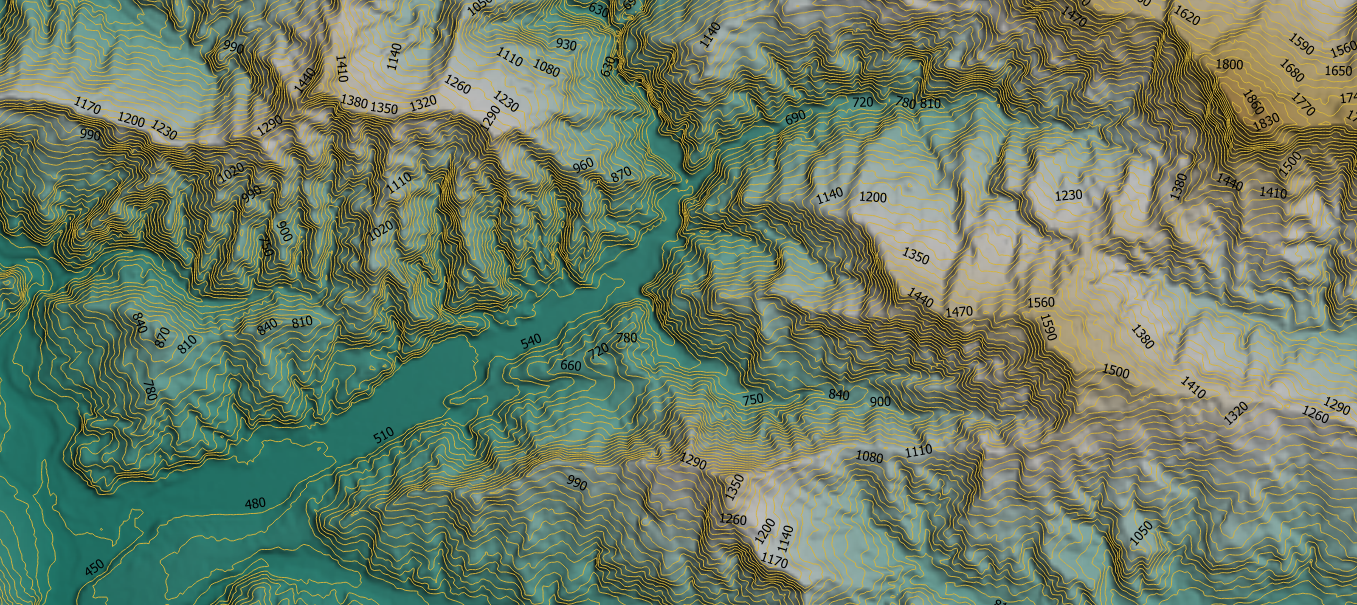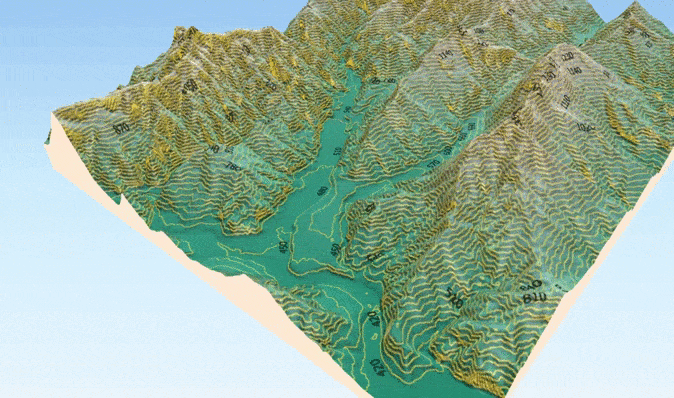# Creating contour lines from STRM and ALOS DEMs on Google Earth Engine

“Contour lines are an important way to show the rise and fall of the terrain on a map. They show all places that are at the same height above sea level. They also tell us about the slope of the terrain and are a layer information base in engineering projects “Contour lines generated from 30 m ALOS PALSAR DEM (Jaxa, 2020) on Google Earth Engine and QGIS platform in a central area of NEPAL

Contour lines are the most distinctive feature of a topographic map. Contour lines are lines drawn on a map that connect points of equal elevation, which means that if you physically followed a contour line, the elevation would remain constant. Contour lines show the elevation and shape of the terrain.Contour lines generated from the 30 m ALOS PALSAR DEM (Jaxa, 2020) on Google Earth Engine in NEPAL

Google Earth Engine is a platform for scientific analysis and visualization of geospatial data sets, for academic, non-profit, commercial and government users. Earth Engine hosts satellite images and stores them in a public data archive that includes historical images of the Earth dating back more than forty years. The Google Earth Engine API provides a library of functions that can generate level lines from the global digital models available from the ALOS and STRM projects.

# Tools

The extraction of contour lines was carried out from the Google Earth Engine platform and QGIS for 3D was visualized, using the Qgis2threejs module.

# Data

For this article, I will be using two datasets. In the case of ALOS, it is necessary to reproject an image tile using a projection of one of the image tiles, rather than using the default projection:

ALOS Global Digital Surface Model “ALOS World 3D of 30m (AW3D30)”

# Basic creation: Option 1

First, we add the repositories of the image collections that contain the DEM (digital terrain model). For ALOS, you should re-project an image tile using a projection of one of the image tiles, rather than using the default projection returned by .mosaic ():

`var dataset = ee.ImageCollection(‘JAXA/ALOS/AW3D30/V3_2’);var elevation = dataset.select(‘DSM’);// projectionvar proj = elevation.first().select(0).projection();var slopeReprojected = (elevation.mosaic().setDefaultProjection(proj));`

Then we reclassify the heights according to the distance between contour lines. (remember that it can never be lower than the resolution of the image). In our case, we classify heights between 0–2000 mamsl:

`// Remap values.var demclass = ee.Image(1)          .where(dem.gt(0).and(dem.lte(500)), 2)          .where(dem.gt(500).and(dem.lte(600)), 3)          .where(dem.gt(600).and(dem.lte(700)), 4)          .where(dem.gt(700).and(dem.lte(800)), 5)          .where(dem.gt(800).and(dem.lte(900)), 6)          .where(dem.gt(900).and(dem.lte(1000)), 7)          .where(dem.gt(1000).and(dem.lte(1100)), 8)          .where(dem.gt(1100).and(dem.lte(1200)), 9)          .where(dem.gt(1200).and(dem.lte(1300)), 10)          .where(dem.gt(1300).and(dem.lte(1400)), 11)          .where(dem.gt(1400).and(dem.lte(1500)), 12)          .where(dem.gt(1500).and(dem.lte(1600)), 13)          .where(dem.gt(1600).and(dem.lte(1700)), 14)          .where(dem.gt(1700).and(dem.lte(1800)), 15)          .where(dem.gt(1800).and(dem.lte(1900)), 16)          .where(dem.gt(1900).and(dem.lte(2000)), 17);          Map.addLayer(demclass.clip(AOI), {min: 1, max: 17, palette: ['black', 'white']}, 'elevationclass ALOS', false);`

Now convert each elevation thresholds to vectors:

`var vectors = zones.addBands(demclass).reduceToVectors({  geometry: AOI,  scale: 30,  geometryType: 'polygon',  eightConnected: false,  labelProperty: 'zone',  reducer: ee.Reducer.mean()});// Add the vectors to the mapvar display = ee.Image(0).updateMask(0).paint(vectors, '000000', 3);Map.addLayer(display, {palette: 'ff0000'}, 'contour lines ALOS', false);`

# Option 2

Instead of reclassifying, use a sequence list to define the height interval and distance between the contour lines:

`var lines = ee.List.sequence(0, 4000, 100);`

Define a function to extract contour lines from a Gaussian kernel and join them:

`var contourlines = lines.map(function(line) {  var mycontour = strmelevation    .convolve(ee.Kernel.gaussian(5, 3))    .subtract(ee.Image.constant(line)).zeroCrossing()     .multiply(ee.Image.constant(line)).toFloat();      return mycontour.mask(mycontour);});contourlines = ee.ImageCollection(contourlines).mosaic();`

Finally, you can export the results as a KML, KMz, or SHP file:

`Export.table.toDrive({  collection: vectors2,  description:'contour lines ALOS',  fileFormat: 'KML'});`

Here in this GitHub repository, you will find the complete code: link